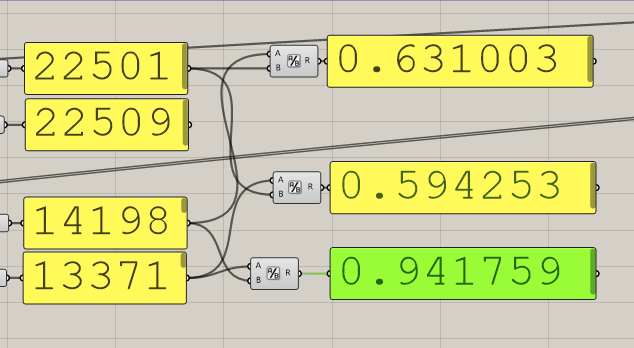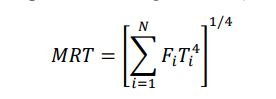# The relationship between annual solar radiation(from radiance) and total solar energy(from energyplus)?

I am working on a research concerning solar radiation and building energy consumption. I found that the E+ can output the total solar energy of one window(beam and diffuse energy). I compared it with the value calculated with radiance, it may be about about 60% of the radiance value. This bias may due to the solar heat gain coefficient (SHGC). But the question raises as I check the results of two different shading settings:
I can build two kind of shading objects that can let the south window achieve the same solar radiation value like this:

(The object A and B have the same effect of shading)
But I notice the total solar energy of the window is different, from this scene, the value of object B is 94% of that of object A. (For other scenes the bias maybe very high )Can I say this difference is because that although Radiance calculated the long wave of solar radiation, it can not calculate that of surrounding surfaces?

I’ve found this article:http://www.ibpsa.org/proceedings/BS2017/BS2017_260.pdf @chris @TheodorosGalanos. If the guess is right, can I use this equation:to make the result closer to the E+ result? I wonder can I do some post-processing to the radiance result to resemble the whole annual solar energy of the E+. This is a part of my research.
Any suggestion is appreciated. Thank you!

For now, I conclude if the shading surface is far enough from the window (like 10meters), the bias will be stay nearly unchanged, for me it is enough.

When comparing solar radiation on windows on E+ and Radiance its hard to make them match due to how they calculate windows. The default “simple window model” in E+ is actually pretty thorough compared to a simple multiplication of SHGC.